# Differential Equations

Also found in: Dictionary, Thesaurus.
Related to Differential Equations: Partial differential equations
The following article is from The Great Soviet Encyclopedia (1979). It might be outdated or ideologically biased.

## Differential Equations

equations containing unknown functions, their derivatives of various orders, and independent variables. The theory of differential equations arose at the end of the 17th century in response to the needs of mechanics and other natural sciences, essentially at the same time as the integral calculus and the differential calculus.

The simplest differential equations are found in the works of I. Newton and G. von Leibniz. The term “differential equation” belongs to Leibniz. While creating the calculus of fluxions and fluents, Newton posed two problems: (1) given a relation between fluents, find the relation between their fluxions and (2) given an equation containing fluxions, find the relation between the fluents. From the modern point of view, the first problem (finding the derivatives of functions) falls under differential calculus, while the second forms the content of the theory of ordinary differential equations. Newton regarded the task of finding the indefinite integral F(x) of the functionf(x) as a special case of his second problem. For Newton, the founder of mathematical natural science, such an approach was wholly justified: in most cases, the laws of nature, which govern various processes, are expressed in the form of differential equations, and the calculation of the course of these processes reduces to the solution of differential equations.

The following two simple examples can serve as an illustration of what has been said.

(1) If a body heated to the temperature T is placed in a medium whose temperature is equal to zero, then under certain conditions we may assume that the increment ΔT (negative when T > 0) of its temperature over a short interval of time Δt can be expressed with sufficient accuracy by the formula

ΔT=-kTΔt

where k is a constant. In the mathematical treatment of this physical problem we assume that the exactly corresponding limit relation between the differentials

(1)dT=-kTdt

holds. In other words, we assume that the differential equation

T=-kT

holds, where T’ denotes the derivative with respect to t. To solve this differential equation, or, as we say, to integrate it, is to find the functions that satisfy it. For equation (1) all such functions (that is, all its particular solutions) have the form

(2)T=Ce-kt

where C is a constant. Formula (2) with an arbitrary constant C is called the general solution of equation (1).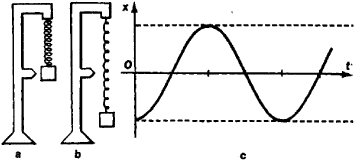Figure 1

(2) Suppose a weight p of mass m attached to a spring is in a state of equilibrium (Figure 1, a). If we stretch the spring (Figure 1, b), then the equilibrium is disturbed and the weight is set in motion. If x(t) denotes the magnitude of the body’s deviation from the state of equilibrium at time t, then the acceleration of the body is expressed by the second derivative x”(t). If the spring is stretched by a small amount, then, according to the theory of elasticity, the force mx”(t) is proportional to the deviation x(t). Thus, one obtains the differential equation

mx”(t) = -kx(t)

Its solution has the form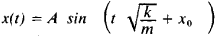and shows that the body will undergo harmonic oscillations (see Figure 1, c).

The theory of differential equations developed into an independent, fully elaborated scientific discipline in the 18th century (the works of D. Bernouilli, J. d’Alembert, and L. Euler).

Differential equations are divided into ordinary differential equations, which involve the derivatives of one or several functions of a single independent variable, and partial differential equations, which involve partial derivatives of functions of several independent variables. The order of the differential equation is the highest order of the derivatives appearing in it. Thus for example,is a partial differential equation of the second order.

Ordinary differential equations, EQUATIONS OF THE FIRST ORDER. The relation

(A) F(x,y,z”) = 0

between the independent variable x, the unknown function y, and its derivative y’ = dy/dx is called an ordinary differential equation of the first order in one unknown function (for the present we will examine only equations of this type). If equation (A) can be solved for the derivative, then we obtain an equation of the form

(B) y’ = f(x,y)

The function f(x, y) is supposed single-valued. It is simpler to examine many questions of the theory of differential equations for such equations.

Equation (B) can be written in the form of a relation between differentials

f(x,y)dx - dy = 0

Then it becomes a particular case of equations of type

(C) P(x,y)dx + Q(x,y)dy = 0

In equations of type (B), it is natural to consider the variables x and y as equivalent, that is, we are not interested in which of them is independent.

GEOMETRIC INTERPRETATION OF DIFFERENTIAL EQUATIONS. Let y = y(x) be a solution of equation (B). In geometric terms this means that in a rectangular coordinate system the slope of the tangent to the curve y = y(x) at each of its points M(x, y) has the value k =f(x, y). Thus, the problem of finding the solution y = y(x) reduces to the following problem: at each point of some domain in the plane we are given a “direction,” and it is necessary to find all curves whose direction at any point M is the same as the preassigned direction at M. If the function f(x, y) is continuous, then this direction changes continuously with M. To give a graphic representation of the preassigned directions, referred to as the direction field, we draw through a sufficiently large number of points distributed with sufficient density over the entire domain under consideration, short dashes with the direction given for these points. In Figure 2 this is done for the equation y1 = y2. The figure enables us to visualize the graphs of the solution curves—the so-called integral curves of the differential equation. Calculation shows that the general solution of this equation is y = 1/(C - x). In Figure 2 the integral curves corresponding to the values of the parameters C = 0 and C = 1 are drawn.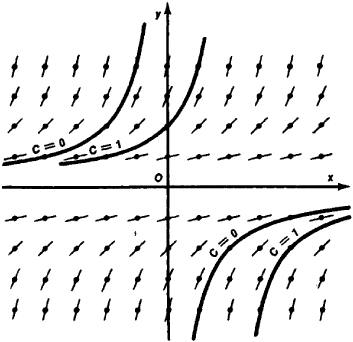Figure 2

The graph of any single-valued function y = y(x) intersects every straight line parallel to the Oy axis only once. Such, consequently, are the integral curves of any equation (B) with a single-valued continuous function on the right-hand side. New possibilities for the form of integral curves arise in connection with equations of type (C). With the aid of the pair of continuous functions P(x, y) and Q(x, y), it is possible to define any continuous direction field. The problem of integrating equations of type (C) coincides with the purely geometric (independent of the choice of coordinate axes) problem of finding the integral curves corresponding to a given direction field in the plane. It should be noted that no definite direction corresponds to the points (x0, y0), at which both functions P(x, y) and Q(x, y) vanish. Such points are called singular points of the equation (C).

For example, consider the equation

ydx + xdy = 0

that can be written in the form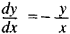Strictly speaking, the right-hand side of the latter equation becomes meaningless for x = 0 andy = 0. The corresponding direction field and the family of integral curves, which in this case are the circles x2 + y2 = C, are shown in Figure 3. The origin (x = 0, y - 0) is a singular point of the differential equation. The integral curves of the equation

ydx = xdy = 0

are depicted in Figure 4. They are the rays from the origin. The origin is a singular point of the equation.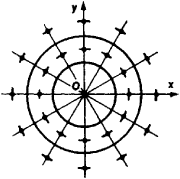Figure 3Figure 4

INITIAL CONDITIONS. The geometric interpretation of differential equations of the first order suggests that through each interior point M of a domain G with a given continuous direction field there passes a unique integral curve.

As regards the existence of an integral curve, the formulated hypothesis is valid. The existence proof was supplied by G. Peano. On the other hand, the uniqueness part of this hypothesis proves, generally speaking, to be incorrect. Even for such a simple equation as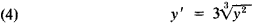whose right-hand side is continuous in the entire plane, the integral curves have the form depicted in Figure 5. Uniqueness of the integral curve passing through a given point is violated at all points of the Ox axis.

Uniqueness, that is, the assertion that there is just one integral curve passing through a given point, holds for equations of type (B) with a continuous right-hand side under the additional assumption that the function/(x, y) has a bounded derivative with respect to y in the domain under consideration.

This requirement is a special case of the following, somewhat broader Lipschitz condition: there exists a constant L such that in the domain under consideration we have the inequality

|f(x,y1) - f(x,y2)|<L|y1 - y2|

This condition is most frequently cited in textbooks as a sufficient condition of uniqueness.

From the analytic point of view, the existence and uniqueness theorems for equations of type (B) signify the following: if the appropriate conditions are fulfilled [for example, the function f(x, y) is continuous and has a bounded derivative with respect to y], then the assignment of an initial value y0 =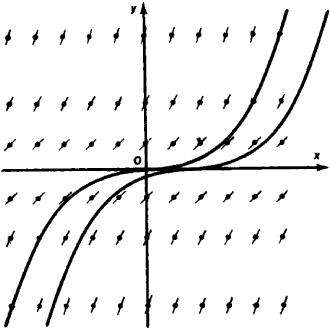Figure 5

y(x0) of the function y(x) for an “initial value” x0 of the independent variable x singles out one definite solution from the family of all solutions y(x). For example, if for equation (1) we require that at the initial time t0 = 0 the temperature of the body be equal to the initial value T0, then we will have singled out a definite solution satisfying the given initial conditions from the infinite family of solutions of (2):

T(t) = T0e- kt

This example is typical: in mechanics and physics differential equations usually determine the general laws of the course of some phenomenon. However, in order to obtain definite quantitative results from these laws, it is necessary to specify data pertaining to the initial state of the physical system being studied at some definite “initial moment” t0.

If the conditions of uniqueness are fulfilled, then the solution y(x) that satisfies the condition y(x0) = y0 can be written in the form

(5) y(x) = Φ(x;x0 +y0)

in which x0 and y0 enter as parameters. The function Φ(x; x0, y0) of the three variables x, x0, and y0 is determined uniquely by equation (B). It is important to note that given a sufficiently small change in the field (the right-hand side of the differential equation), the function Φ x0, y0) changes arbitrarily little over some finite interval as x varies—in other words, there is a continuous dependence of the solution on the right-hand side of the differential equation. If the right-handFigure 6

side f(x, y) of the differential equation is continuous and its derivative with respect to y is bounded (or satisfies a Lipschitz condition), then Φ(x; x0, y0) is again continuous with respect to x0 and y0.

If the conditions of uniqueness for equation (B) are satisfied in a neighborhood of the point (x0, yj, then all the integral curves passing through a sufficiently small neighborhood of the point (x0, y0) intersect the vertical line x = x0 and each of them is determined by the ordinate y = C of its point of intersection with this line (see Figure 6). Thus, all these solutions belong to the family with the single parameter C:

yx = F(x,C)

which is the general solution of the differential equation (B).

In the neighborhood of points at which the conditions of uniqueness are violated, the picture can be more complex. The question of the behavior of the integral curves “in the large” rather than in the neighborhood of the point (x0, y0) is also quite complex.

GENERAL INTEGRAL. SINGULAR SOLUTIONS. It is natural to pose the converse problem: given a family of curves depending on a parameter C, find a differential equation for which the curves of the given family would serve as integral curves. The general method of solving this problem consists in the following. Considering the family of curves in the xOy plane to be defined by the relation

(6) F(x,y,C) = 0

we differentiate (6) keeping C constant and obtain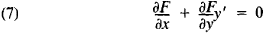or in symmetric notation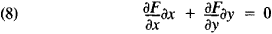and eliminate the parameter C from the two equations (6) and (7) or (6) and (8). If a differential equation is obtained from the relation (6) in this manner, then this relation is called the general integral of the differential equation. The same differential equation can have many different general integrals. After finding the general integral for a given differential equation, it still proves necessary, generally speaking, to check whether the differential equation has additional solutions not contained in the family of integral curves (6).

Let, for example, the family of curves

(9) (x - C)3 - y = 0

be given. If we keep C constant and differentiate (9), then we obtain

3(x - c)2 - y’ = 0

After elimination of C we arrive at the differential equation

(10) 27y2 - (y’)3 = 0

which is equivalent to equation (4). It is easy to see that, in addition to the solutions (9), equation (10) has the solution

(11) y ≡ 0

The most general solution of equation (10) is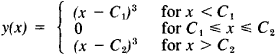where –∞ ≤ C1C2 ≤ +∞ (Figure 7). This solution depends on the two parameters C1 and C2 but is formed from segments of curves of the one-parameter family (9) and a segment of the singular solution (11).

Solution (11) of equation (10) can serve as an example of a singular solution of a differential equation. As another example we examine the family of lines

(12) 4(y - Cx) + C2 = 0

These lines are integral curves of the differential equation

4(y - xy’) + (y’)2 = 0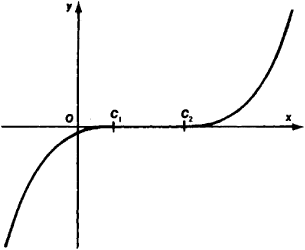Figure 7

A singular integral curve of this differential equation is the parabola

x2 - y = 0

which is the envelope of the lines (12) (Figure 8). This situation is typical: singular integral curves are usually envelopes of the family of integral curves of the general solution.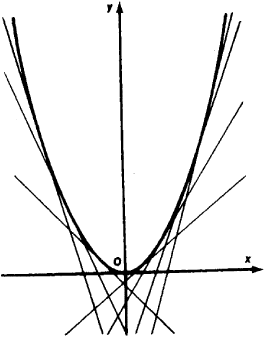Figure 8

DIFFERENTIAL EQUATIONS OF HIGHER ORDER AND SYSTEMS OF DIFFERENTIAL EQUATIONS. Differential equations of the nth order in one unknown function y(x) of the independent variable x are written as follows:If one introduces the additional unknown functions

(14) y1 = y1,y2 = y’’,…yn - 1 = y(n 1)

then equation (13) can be replaced by a system of n equations of the first order in n unknown functions. For this it is sufficient to add to the n-1 equations (14) the equation

F(x, y, y1, y2, . . . , yn - n, yn - n) = 0

A system of equations of higher order can also be reduced to a system of equations of the first order in an analogous manner. In mechanics the reduction of a system of equations of the second order to a system of twice as many equations of the first order has a simple mechanical meaning. For example, by introducing the components u, v, and w of velocity as new variables we can reduce the system of three equations of the motion of a material point

mxn = P(x,y,z)

myn = Q(x,y,z)

mzn = R(x,y,z)

where x, y, and z are the coordinates of a point dependent on time /, to a system of six equations:

mu’ = P(x,y,z)

mv’ = Q(x,y,z)

mw’ = R(x,y,z)

u = x’

v = y’

w = z’

Of greatest value are the systems in which the number of equations is equal to the number of unknown functions. A system of n equations of the first order in n unknown functions, which is solved with respect to the derivatives, has the form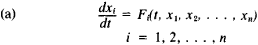A solution of the system of differential equations (a) is a system of functions x1, x£t), … , xn(t), which, when substituted in the equations (a), satisfies them. One frequently encounters systems of type (a), in which the right-hand sides do not depend on f. In this case, the study of the system (a) essentially reduces to the study of the system of the (n-l) equations, which it is advisable to write in the symmetric form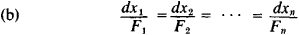without predetermining on which of the variables xl x2, … , xn the remaining n - 1 variables are supposed to depend. By considering x = (x1 x2, … , xn) as a vector, it is possible to write the system (a) in the form of one vector equation: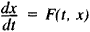which allows extensive use of the analogy with the theory of one equation of the first order of type (B). In particular, it turns out that for the system (a) the basic results concerning the existence and uniqueness of the solution of the initial value problem remain in force: if in a neighborhood of the point (t0, x1°, x2°, … , -xn°) all functions Ft are continuous in all the variables t, x1x2, … , xn and have bounded derivatives with respect to the variables x1x2, xn, then the assignment of the initial values xi(t0) = xi0, for i = 1, 2, … , n, determines a unique solution of the system (a). This explains the fact that, generally speaking, the solution of a system of n equations of the first order in n unknown functions depends on n parameters.

In the case of the above-cited specific examples of differential equations, the general solution can be expressed in terms of elementary functions. The classes of differential equations which admit this type of solution have been studied in detail. Often a more general point of view is adopted, namely, we consider a differential equation “solved,” if the required connection between the variables (and the parameters c1, c2, … , which are a part of the general solution) can be expressed in terms of elementary functions and their integrals (“the solution is expressed in quadratures”).

A general method for finding solutions of differential equations is expansion in power series. For example, if the right-hand sides of equations of type (a) are holomorphic in a neighborhood of the point (t0, x1°, x2°, … , xn°), then the solution of the corresponding initial value problem is given by functions x£t), which can be expanded in power seriesthe coefficients of which can be determined by sucessively differentiating the right-hand sides of the differential equations (a) and by equating the coefficients of the same powers on both sides of these equations.

When it comes to special types of differential equations, there is a very extensive theory of linear differential equations and of systems of linear differential equations.

For linear differential equations it is also relatively easy to solve questions to the “qualitative” behaviors of integral curves, that is, their behaviors in the entire domain of definition of the differential equations. For nonlinear differential equations, for which it is especially difficult to find a general solution, questions of the qualitative theory of differential equations sometimes assume a dominant significance. Following the classical works of A. M. Liapunov, the works of Soviet mathematicians, physicists, and scientists in the field of mechanics have played a leading role in the qualitative theory of differential equations.

Of great importance is the analytic theory of differential equations, which studies the solutions of differential equations from the point of view of the theory of analytic functions. An example of a concern in this theory is the distribution of the singular points of solution functions in the complex plane.

In addition to initial value problems, where the values of the unknown functions are given (and, in the case of equations of a higher order, also their derivatives) at one point (for one value of the independent variable), one frequently makes use of boundary value problems.

Partial differential equations. A typical feature of partial differential equations and of systems of partial differential equations is that in order to determine a particular solution it is necessary to prescribe certain functions rather than the values of a finite number of parameters. For example, the general solution of the equation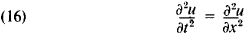is given by any function of the form

u(t,x) = f(x + t) + g(x - t)

where/and g are arbitrary functions. Thus, the differential equation (16) limits the arbitrariness in the selection of a function of two variables u(x, y) only to the extent that it is possible to express it in terms of two functions/f(z) and g(v) of one variable, which remain arbitrary [provided that equation (16) is not supplemented by “initial” or “boundary” conditions] .

The so-called Cauchy problem can serve as a typical problem with initial conditions for a system of partial differential equations of the first orderwhere t, x1… ,xn are the independent variables and U1, … , um are functions of these independent variables. The Cauchy problem consists in the following: to find the functions ui(t, x1… ,xn) given their values for some t = t0:

ut(t0,x1…xn)=Φ(x1,…xn)
i=1,2,…,m

The theory of partial differential equations of order higher than the first and of systems of partial differential equations examines problems of the Cauchy type as well as a number of boundary value problems.

The type of equation is of particular importance in stating and solving boundary value problems for partial differential equations of order higher than the first. As an example we can cite the classification of partial differential equations of the second order in one unknown function z(x, y) of two variables:

(18) F(x, y, z, p, q, r, s, t) = 0

where If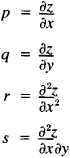then (18) is an elliptic equation. An example of an elliptic equation is the Laplace equation: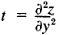If D < 0, then (18) is a hyperbolic equation. An example of a hyperbolic equation is the equation of vibrating string: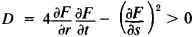If D = 0, then (18) is a parabolic equation. An example of a parabolic equation is the heat equation: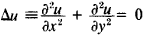### REFERENCES

Ordinary differential equations
Stepanov, V. V. Kurs differentsial’nykh uravnenii, 8th ed. Moscow, 1959.
Petrovskii, I. G. Lektsii po teorii obyknovennykh differentsial’nykh uravnenii, 5th ed. Moscow, 1964.
Pontriagin, L. S. Obyknovennye differentsial’nye uravneniia, 2nd ed. Moscow, 1965.
Kamke, E. Spravochnik po obyknovennym differentsial’nym uravneniiam, 3rd ed. Moscow, 1965. (Translated from German.)
Filippov, A. F. Sbornik zadach po differentsial’nym uravneniiam, 2nd ed. Moscow, 1965.
Differential equations with partial derivatives
Petrovskii, I. G. Lektsii ob uravneniiakh s chastnymi proizvodnymi, 3rd ed. Moscow, 1961.
Tikhonov, A. N., and A. A. Samarskii. Uravneniia matematicheskoi fiziki, 3rd ed. Moscow, 1966.
Sobolev, S. L. Uravneniia matematiceskoi fiziki, 4th ed. Moscow, 1966.
Smirnov, M. M. Zadachi po uravneniiam matematicheskoi fiziki, 5th ed. Moscow, 1968.
(On the basis of the article of the same title from the 2nd edition of the Great Soviet Encyclopedia)
References in periodicals archive ?
The linearization problem of third-order ordinary differential equations under point transformations was solved by Bocharov et al.
Poole, Introduction to The Theory of Linear Differential Equations, vol.
(2) In the paper titled "Approximate Controllability for Functional Equations with Riemann-Liouville Derivative by Iterative and Approximate Method," the authors discussed the functional control systems governed by differential equations with Riemann-Liouville fractional derivative in general Banach spaces.
However, here, we would only like to summarize some works that can be found in the literature and methods used during the investigations of the existence of the periodic solutions, stability, asymptotic stability, square integrability, and boundedness of solutions of ordinary and functional differential equations of second order.
This paper has also a contribution to the subject in the literature, and it may be useful for researchers working on the qualitative behaviors of solutions to Volterra integro- differential equations. In view of all the mentioned information, it can be checked the novelty and originality of the present paper.
Over the years many scientific works have been dedicated to the mentioned problems for various differential equations, fractional differential equations, partially differential equations, and so forth.
This is very similar to the theory of crisp stochastic differential equations. However, unlike crisp equations, fuzzy set-valued equations exhibit new qualitative properties of their solutions.
Although second-order stochastic delay differential equations have started receiving attention of authors, according to our observation from relevant literature, there is no previous literature available on the stability and boundedness of solutions of second-order nonlinear nonautonomous stochastic differential equation.
Since, partial differential equations in the coupled problem under consideration had complicated mixed partial derivatives (Handibag and Karande, 2012).
Improvement in technology makes it much easier to solve a huge system of differential equations numeric ally.
It is a supplement for elementary and intermediate courses in differential equations, providing over 900 problems to practice on.
During the last few years, the numerical methods and exact solution methods have been proposed to solve fractional differential equations, for example, the Adomian decomposition method , the homotopy perturbation method [11, 12], the variational iteration method [13, 14], the differential transform method [15,16], the G'/G method [17, 18], the first integral method , and the exp-function method .

Site: Follow: Share:
Open / Close